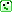Some excellent responses! Just one small issue, coming from some mistakes in _Seven Sketches_. Everything Matthew and Owen just said is true for posets, but not for preorders.

Remember that a **preorder** is a set with a binary relation \\(\le\\) that's reflexive and transitive. A **poset** is a preorder where \\(x \le y\\) and \\(y \le x\\) imply \\(x = y\\).

The left or right adjoint of a monotone function between posets is unique if it exists. This need not be true for preorders.

The issue can be seen clearly in the phrases "the smallest \\(b\\) with \\(a \le g(b)\\)". In a poset, such an \\(a\\) is unique if it exists. In a preorder, that's not true, since we could have \\(a \le a'\\) and \\(a' \le a\\) yet still \\(a \ne a'\\).

Adding to the confusion, _Seven Sketches_ uses "poset" to mean "preorder", and "skeletal poset" to mean "poset". So, when the authors say the left or right adjoint of a monotone function between posets is unique if it exists, that's true with the _usual_ definition of poset, but not for _their_ definition.Luckily, I have convinced the authors to straighten this out. Here's what I wrote in an email to Brendan Fong.
He just replied saying that he and David are fixing the mistakes I describe, and switching to the standard definition of "poset".

Someone in the course pointed out something that's more than a typo. If you're going to use "poset" to mean "preorder" (bad, bad, bad) then you can't talk about "the" meet or join of two elements in a poset, because even when it exists it's not unique.

Of course you can use "the" in the sophisticated way, meaning "unique up to canonical isomorphism"... but that seems a bit fancy for your intended audience, and it at least would need to be explained.

You guys just say things like:

> Let P be a poset, and let A be a subset. We say that an element is the meet of A if ...

You could fix this by changing "the" to "a", but every equation you write down involving meets and joins is wrong unless you restrict to the "skeletal poset" case. For example, Example 1.62:

> In any poset P, we have \\(p \vee p = p \wedge p = p\\).

More importantly, Prop. 1.84 - right adjoints preserve meets. The equations here are really just isomorphisms!

This then makes your statement of the adjoint functor theorem for posets incorrect.

I think this is the best solution:

1. Call preorders "preorders" and call posets "posets". Do not breed a crew of students who use these words in nonstandard ways! You won't breed enough of them to take over the world, so all you will accomplish is making them less able to communicate with other people. And for what: just because you don't like the sound of the word "preorder"?

2. Define meets and joins for preorders, but point out that they're unique for posets, and say this makes things a bit less messy.

3. State the adjoint functor theorem for posets... actual posets!